# Spreadsheets are not just for financial experts and accountants. They can also be suitable for freelancers or small business owners like you.

Spreadsheets can help record key information about jobs, study bestsellers, and organize people’s lives.

The main purpose of Excel is to make your life easier. This article will help you develop the basic skills to work with Excel. Formulas are the core of Excel.

• How to write your first formula in Microsoft Excel for math automation.
• How to copy and paste the current formula into another cell.
• And How to use autofill to quickly use formulas across an entire column.

You can see why Excel is so powerful by looking at the spreadsheet below. The top image shows the behind-the-scenes formulas, while the bottom image shows what the formulas offer.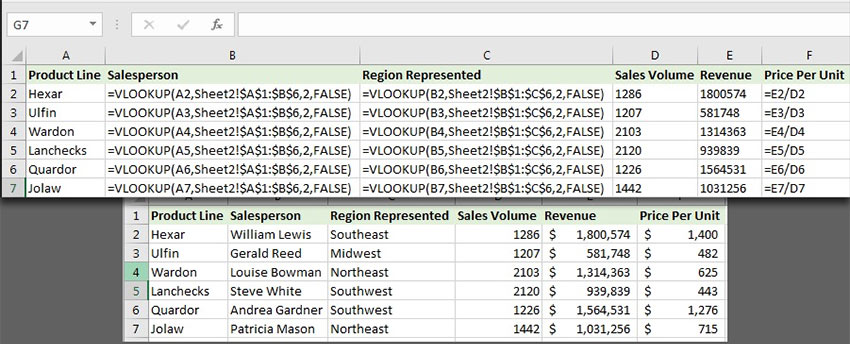Spreadsheets simplify data by using formulas to calculate and modify.

In this section, we write our first formula in Microsoft Excel. Formulas must be written inside cells, which are arranged like separate boxes on a spreadsheet. Excel is a huge and continuous network of rows and columns. Where rows and columns meet is called a cell.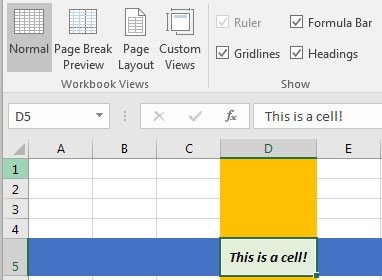A cell is formed when rows (numbered boxes on the left) and columns (lattice boxes from left to right) intersect.

Cells are where you can type data or formulas that work with your data. Each cell has a name that you can use when talking about spreadsheets.

Rows are horizontal boxes numbered on the left. The columns run from left to right and are named with letters. When rows and columns meet, they form a cell. The intersection of column C and row 3 is a cell called C3.

In the image below, I have manually typed the names of the cells in Excel to help you understand how they work.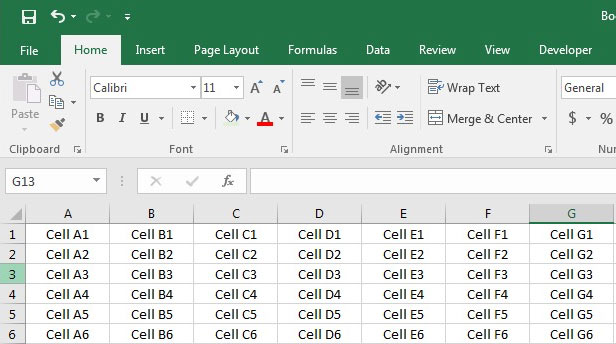The cells are named as the junction of rows and columns. It consists of the intersection of a column F and a 4-cell row, which we call F4.

Now that you understand the structure of a spreadsheet in Excel, we can start writing formulas and functions in Excel.

## How to work with Excel formulas and functions

Formulas and functions are what we use to work with typed data. Excel provides internal functions such as AVERAGE = to calculate the average of numbers, formulas are actually simple functions such as adding two cells to each other. These two terms can have the same meaning.

In this article, I will use the term formula to refer to these because we often work with a combination of both on a spreadsheet.

To write your first formula, double-click on a cell and type =. Let’s use a simple example as the first formula: like adding two values ​​together.

= 4 + 4

This formula appears as follows in Excel: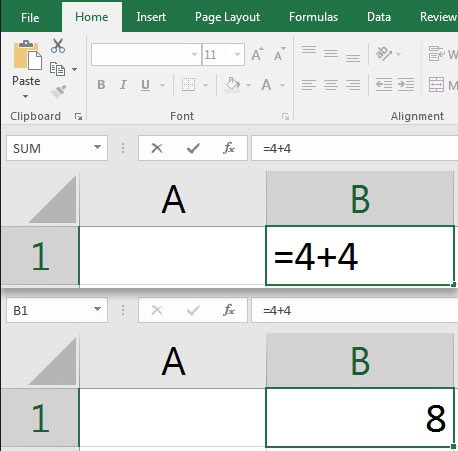A simple formula and its result in Excel.

By pressing Enter on the keyboard, Excel will evaluate what you typed. It actually calculates the formula you typed and displays the result. When we add two values ​​to each other, Excel calculates their sum and prints the total value.

Notice the formula bar at the top of your spreadsheet that shows the formula for adding two numbers. This formula stays behind the scenes when the result is displayed.

The cell containing   “= 4 + 4” is considered as a formula, while the spreadsheet prints the result. Using similar formulas such as subtraction, multiplication (with *) and division (with symbol), try typing formulas in the formula bar or in a cell.

This seemingly simple example illustrates an important concept: Behind your spreadsheet cells, you can create powerful formulas that work with your data.

## Excel formulas with cellular references

There are two ways to work with these formulas:

• Use the data in the formulas as in the example above (4 + 4 =)
• For convenience, use formulas with cell references, such as A2 + A3 =

See the example below. In the picture below, I have entered the sales list of the company in the first 3 months of 2016. Now I add the sales of these three months together.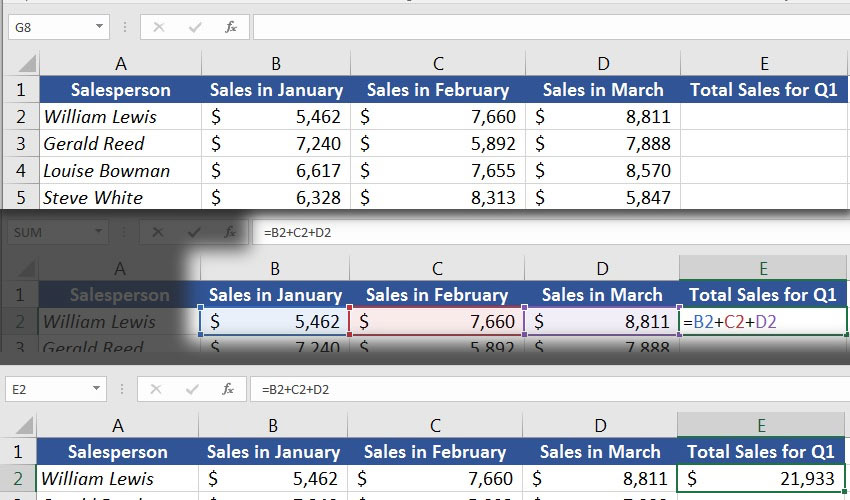In this example, I use an inline formula with cell reference. I added cells B2, C2, and D2 together to calculate total sales for the first three months of the year.

The formulas we have worked with so far are just a few of the total formulas available in Excel. You can also try the following formulas:

• AVERAGE = To calculate the average.
• COUNT = To count the number of items in a data set.
• TODAY = To print today’s date in a cell.
• TRIM = to remove empty spaces from the beginning and end of a cell.

## How to copy and paste formulas in Excel

After learning how to write formulas, we also need to know how to copy and paste them.

When we copy and paste a cell with its formula, the cell value is not copied – but the formula is copied. If we paste it elsewhere, we actually enter the formula elsewhere.

In the following example, you can see the steps I did:

1. I copied cell E2 (Ctrl + C) (which is a formula that combines B2, C2 and D2).
2. Then, by clicking and dragging the column, I selected the other cells in column E.
3. I pressed Ctrl + V) to paste the same formula into all cells in column E.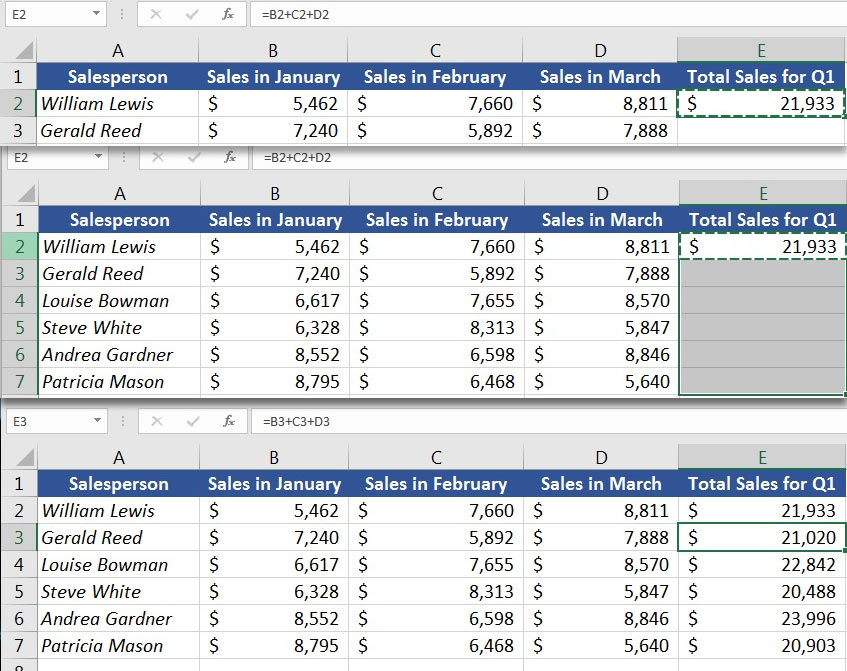When you copy and paste a formula in Excel, its value is not copied – but the formula (in other cells) is copied.

As you can see in the image above, the value is not inserted by pasting the formula (\$ 21933). Rather, the formula itself is copied.

The formula we copied was in cell E2. And gathered cells B2, C2 and D2 together. When we attached it to cell E3, it did not do the same thing – but added the values ​​B3, C3 and D3 together.

In fact, Excel thinks you want to add the three cells to the left of the current cell together. When you copy and paste a formula, it will copy relative references instead of copying and pasting the same references.

## How to Autofill Formulas in Excel

Autofill Formulas is one of the fastest ways to expand your formulas. If all the cells in which you want to copy your formula are adjacent to each other, you can autofill the formulas on your spreadsheet.

For autofill in Excel, scroll through the cell with your formula. By placing the cursor in the lower right corner, the cursor will look like a plus sign. To autofill formulas, just double-click.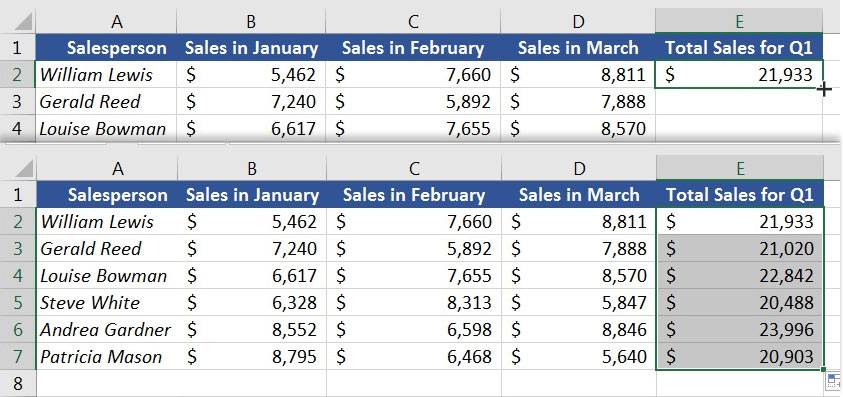Rotate the cursor to the bottom right corner of a cell to display a “+” sign, then double-click to autofill formulas.

Note: One thing to keep in mind when using autofill is that Excel can not always guess the correct formulas. So you need to make sure that autofill puts the right formulas on your spreadsheet.

## Relative references versus absolute references

Let’s make the discussion more specialized in this section and talk about relative references versus absolute references in Excel.

In the example where we calculated the sales amount for the first quarter of the year, we wrote a formula with relative references. That’s why it worked right when we pulled the formula down. Because instead of adding those three cells, he put the three cells on the left side of the cell together.

Absolute references freeze the cells to which we refer. In the following example, we try to show that absolute references are also useful.

In this section, we want to calculate the sales bonus of our employees in the first three months of the year. Based on the sales made, we calculate a sales bonus of 2.5%. Let’s start by multiplying the number of sales by the percentage of rewards for the first employee.

#### Assign a box to the bonus percentage, and multiply the number of sales by the bonus percentage: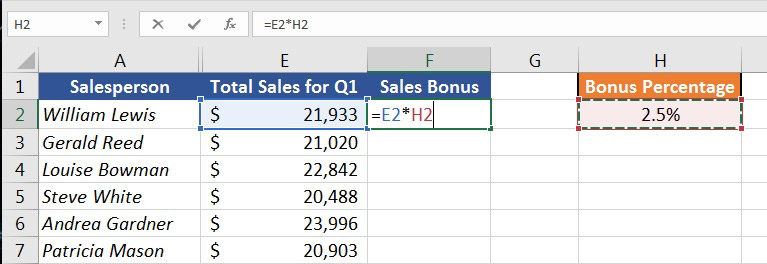We have written a formula for multiplying the number of sales by the percentage of rewards.

We have now calculated the first selling bonus, so we pull down the formula to calculate the other bonuses: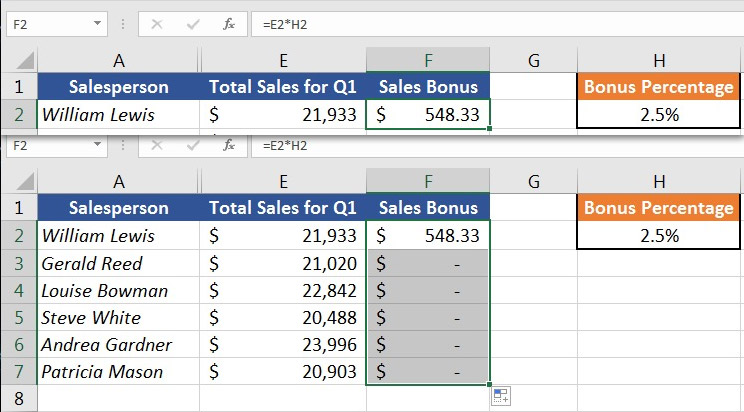Although the calculation of the first sales bonus went well, the formula was not responsive to the calculation of other bonuses.

Unfortunately, the formula did not work. Since the reward percentage always remains in the cell – H2; So it does not work when we pull the formula down. And we get the number zero in the cells.

#### Here’s how Excel works to calculate F3 and F4: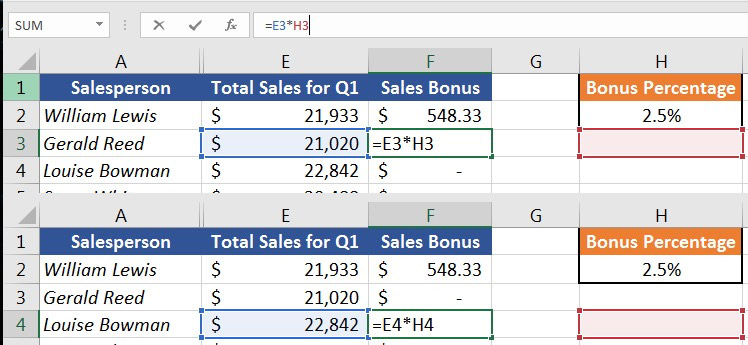Our formula needs an absolute reference to multiply in cell H2 instead of being stretched.

In fact, we need to change the formula so that Excel uses the H2 cell to multiply the reward. This is where we need to use an absolute reference.

Absolute references tell Excel to freeze the cell used in the formula and not change it by changing the formula.

So far, our formula has been as follows:

= E2 * H2

#### To freeze this formula to use the H2 cell, we need to make it an absolute reference:

= E2 * \$ H \$ 2

Note that we add the dollar sign in the cell reference. This gives Excel the message that no matter which cell we put our formula in, it must go to cell H2 to use the reward percentage. We leave E2 unchanged because when we pull down the formula, we want it to be compatible with selling all employees.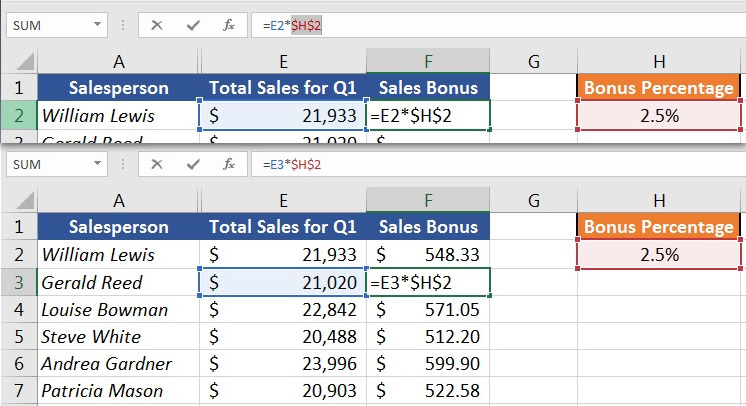Absolute references allow us to lock the cell in the formula even when we pull the formula down.

Absolute references allow you to specify how your formulas work. In this example, we used an absolute reference to lock the formula to the same reward percentage for all employees.

Formulas make Excel powerful. If you do not want to do things manually on your spreadsheet, just write a formula and drag it.